﻿ Common Core Standards - MathAdaptive Worksheets

Helping Every Child to Succeed

toll-free (888) 777-0876
fax (888) 777-0875

## Operations and Algebraic Thinking for Grade 1

### Represent and solve problems involving addition and subtraction.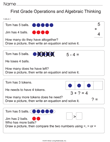Quick Assessment for 1.OA.1 and 1.OA.2
• 1.OA.1. Use addition and subtraction within 20 to solve word problems involving situations of adding to, taking from, putting together, taking apart, and comparing, with unknowns in all positions, e.g., by using objects, drawings, and equations with a symbol for the unknown number to represent the problem.1
##### Subtraction Word Problems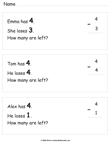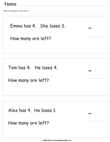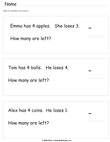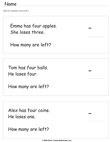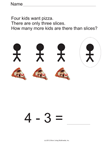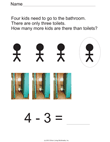• 1.OA.2. Solve word problems that call for addition of three whole numbers whose sum is less than or equal to 20, e.g., by using objects, drawings, and equations with a symbol for the unknown number to represent the problem.

### Understand and apply properties of operations and the relationship between addition and subtraction.

• 1.OA.3. Apply properties of operations as strategies to add and subtract.2 Examples: If 8 + 3 = 11 is known, then 3 + 8 = 11 is also known. (Commutative property of addition.) To add 2 + 6 + 4, the second two numbers can be added to make a ten, so 2 + 6 + 4 = 2 + 10 = 12. (Associative property of addition.)
• 1.OA.4.Understand subtraction as an unknown-addend problem. For example, subtract 10 – 8 by finding the number that makes 10 when added to 8. Add and subtract within 20.

### Add and subtract within 20.

• 1.OA.5. Relate counting to addition and subtraction (e.g., by counting on 2 to add 2).
• 1.OA.6. Add and subtract within 20, demonstrating fluency for addition and subtraction within 10. Use strategies such as counting on; making ten (e.g., 8 + 6 = 8 + 2 + 4 = 10 + 4 = 14); decomposing a number leading to a ten (e.g., 13 – 4 = 13 – 3 – 1 = 10 – 1 = 9); using the relationship between addition and subtraction (e.g., knowing that 8 + 4 = 12, one knows 12 – 8 = 4); and creating equivalent but easier or known sums (e.g., adding 6 + 7 by creating the known equivalent 6 + 6 + 1 = 12 + 1 = 13).

### Work with addition and subtraction equations.

• 1.OA.7. Understand the meaning of the equal sign, and determine if equations involving addition and subtraction are true or false. For example, which of the following equations are true and which are false? 6 = 6, 7 = 8 – 1, 5 + 2 = 2 + 5, 4 + 1 = 5 + 2.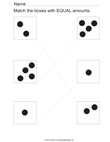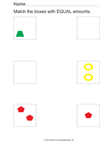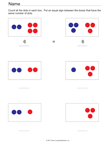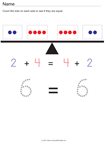• 1.OA.8. Determine the unknown whole number in an addition or subtraction equation relating three whole numbers. For example, determine the unknown number that makes the equation true in each of the equations 8 + ? = 11, 5 = _ – 3, 6 + 6 = _.

The Common Core State Standards are © Copyright 2010. National Governors Association Center for Best Practices and Council of Chief State School Officers. All rights reserved. For more information about the Common Core State Standards, visit their website at http://www.corestandards.org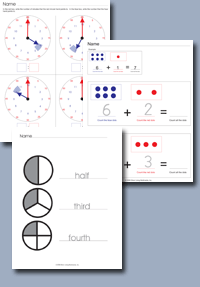Click on any of the standards on the left to create custom worksheets or to print premade materials.

The Common Core State Standards are © Copyright 2010. National Governors Association Center for Best Practices and Council of Chief State School Officers. All rights reserved. For more information about the Common Core State Standards, visit their website at http://www.corestandards.org